# MCAT Physics Practice Test 1: Kinematics and Dynamics

### Test Information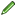15 questions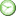minutes

Take more free MCAT physics practice tests available from maintests.com.

1. A man walks 30 m east and then 40 m north. What is the difference between his traveled distance and his displacement?

• A. 0 m
• B. 20 m
• C. 50 m
• D. 70 m

2. A 1000 kg rocket ship, travelling at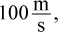is acted upon by an average force of 20 kN applied in the direction of its motion for 8 s. What is the change in velocity of the rocket?

• A.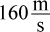• B.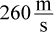• C.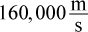• D.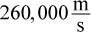3. A car is traveling at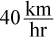and the driver puts on the brakes, bringing the car to rest in a time of 6 s. What is the magnitude of the average acceleration of the car?

• A.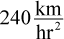• B.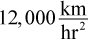• C.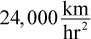• D.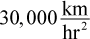4. An elevator is designed to carry a maximum weight of 9800 N (including its own weight), and to move upward at a speed of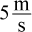after an initial period of acceleration. What is the relationship between the maximum tension in the elevator cable and the maximum weight of the elevator while the elevator is accelerating upward?

• A. The tension is greater than 9800 N.
• B. The tension is less than 9800 N.
• C. The tension equals 9800 N.
• D. It cannot be determined from the information given.

5. At a place where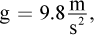an object is thrown vertically downward with a speed of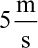while a different object is thrown vertically upward with a speed of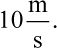Which object undergoes a greater change in speed in a time of 2 s?

• A. The first object has a greater change in speed.
• B. The second object has a greater change in speed.
• C. Both objects undergo the same change in speed.
• D. It cannot be determined from the information given.

6. A firefighter jumps horizontally from a burning building with an initial speed of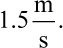At what time is the angle between his velocity and acceleration vectors the greatest?

• A. The instant he jumps
• B. When he reaches terminal velocity
• C. Halfway through his fall
• D. Right before he lands on the ground

7. A 10 kg wagon rests on a frictionless inclined plane. The plane makes an angle of 30° with the horizontal. Approximately how large is the force required to keep the wagon from sliding down the plane?

• A. 10 N
• B. 49 N
• C. 85 N
• D. 98 N

8. Which of the following expressions correctly illustrates the SI base units for each of the variables in the formula below?

mΔv = FΔt

• A. lb × mph = ft × lb × s
• B.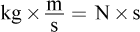• C.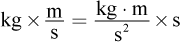• D.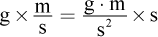9. A 20 kg wagon is released from rest from the top of a 15 m long lane, which is angled at 30° with the horizontal. Assuming that there is friction between the ramp and the wagon, how is this frictional force affected if the angle of the incline is increased?

• A. The frictional force increases.
• B. The frictional force decreases.
• C. The frictional force remains the same.
• D. It cannot be determined from the information given.

10. Which of the following quantities is NOT a vector?

• A. Velocity
• B. Force
• C. Displacement
• D. Distance

11. A 30 kg girl sits on a seesaw at a distance of 2 m from the fulcrum. Where must her father sit to balance the seesaw if he has a mass of 90 kg?

• A. 67 cm from the girl
• B. 67 cm from the fulcrum
• C. 133 cm from the girl
• D. 267 cm from the fulcrum

12. A BASE jumper runs off a cliff with a speed of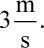Which of the following is closest to his speed after 0.5 seconds?

• A.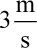• B.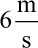• C.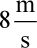• D.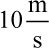13. A rock (m = 2 kg) is shot up vertically at the same time that a ball (m = 0.5 kg) is projected horizontally. If both start from the same height:

• A. the rock and ball will reach the ground at the same time.
• B. the rock will reach the ground first.
• C. the ball will reach the ground first.
• D. the rock and ball will collide in the air before reaching the ground.

14. Centrifugal force is an apparent outward force during circular motion. It has been described as a reaction force according to Newton's third law. Which of the following statements is most likely to be correct regarding centrifugal force?

• A. Centrifugal force exists only for uniform circular motion, not nonuniform circular motion.
• B. Centrifugal force exists only when tension or a normal force provides centripetal acceleration.
• C. Centrifugal force always acts antiparallel to the centripetal force vector.
• D. Centrifugal force is result of repulsive electrostatic interactions.

15. Which of the following statements is true of movement on a plane with friction?

I. Acceleration is a function of applied force only.

II. More force is needed to accelerate a stationary object than a moving object.

III. The force of friction is independent of the mass of objects.

• A. I only
• B. II only
• C. I and II only
• D. I and III only

﻿